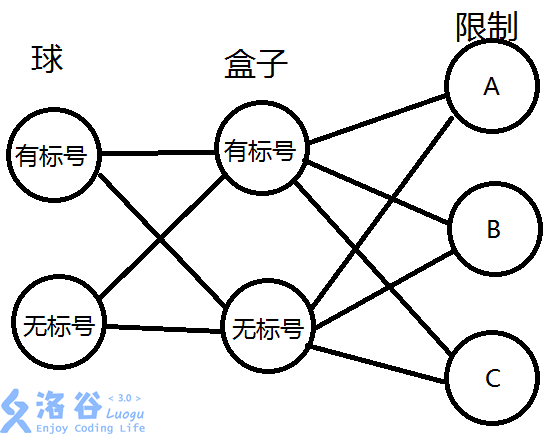# 组合数学入门—TwelveFold Way

$n$个有标号/无标号的球分给$m$个有标号/无标号的盒子

A、无限制

B、每个盒子至少有一个球

C、每个盒子至多有一个球### LLB

$ans = \sum_{i = 0} ^ m (-1) ^ i \binom{n}{i} (m - i) ^ n$

### LLC

$\binom{m}{n}\times n!$

### LUB

$S_{n}^m = S_{n - 1}^{m - 1}+ m\times S_{n - 1}^m$

### LUA

$ans = \sum_{i = 1}^mS_{n}^i$

### ULB

$x_1+x_2+x_3+\dots +x_m = n$

$\binom{n - 1}{m - 1}$

### ULA

$y_i = x_i+ 1$

$y_1+y_2+\dots+y_m = n+m$

$\binom{n + m - 1}{m - 1}$

### UUB

$P_{i.j} = P_{i - 1,j - 1} + P_{i - j,j}$

### UUA

$ans = \sum_{i = 1}^m P_n^i$

posted @ 2019-10-18 08:02  wyxdrqcccc  阅读(...)  评论(...编辑  收藏